Courses

# Redox Reactions (Chapter Test - Medical)

## 20 Questions MCQ Test | Redox Reactions (Chapter Test - Medical)

Description
This mock test of Redox Reactions (Chapter Test - Medical) for Class 12 helps you for every Class 12 entrance exam. This contains 20 Multiple Choice Questions for Class 12 Redox Reactions (Chapter Test - Medical) (mcq) to study with solutions a complete question bank. The solved questions answers in this Redox Reactions (Chapter Test - Medical) quiz give you a good mix of easy questions and tough questions. Class 12 students definitely take this Redox Reactions (Chapter Test - Medical) exercise for a better result in the exam. You can find other Redox Reactions (Chapter Test - Medical) extra questions, long questions & short questions for Class 12 on EduRev as well by searching above.
QUESTION: 1

Solution:
QUESTION: 2

Solution:
QUESTION: 3

### The reaction,  shows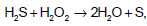Solution:
QUESTION: 4

In which of the following reactions, the underlined substance is oxidised ?

Solution:
QUESTION: 5

In the reaction,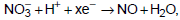the value of x is

Solution:
QUESTION: 6

Which of the following statements is correct ?

Solution:
QUESTION: 7

In the redox reaction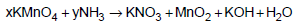Solution:
QUESTION: 8

The oxidation state of ‘S’ in H2S2O8 is

Solution: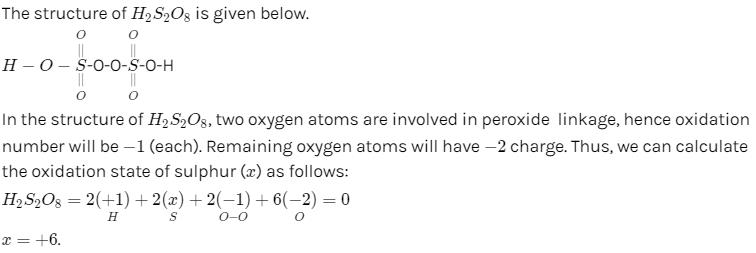QUESTION: 9

The reaction of calcium with water is represented by the equation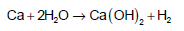What volume of H2 at STP would be liberated when 8 g of calcium completely reacts with water ?

Solution:
QUESTION: 10

In the balanced chemical reaction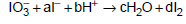a, b, c and d respectively correspond to

Solution:
QUESTION: 11

In which of the following reaction H2O2 does not act as oxidising agent ?

Solution:
QUESTION: 12

The oxidation number of phosphorous in Mg2P2O7 is

Solution:
QUESTION: 13

For reducing one mole of Fe2+ ion to Fe, the number of faraday of electricity is

Solution:
QUESTION: 14

If HNO3 changes to N2O, the oxidation number is changed by

Solution:
QUESTION: 15

Which of the following have been arranged in order of decreasing oxidation number of sulphur ?

Solution:
QUESTION: 16

Given :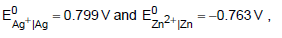Which of the following fact is correct ?

Solution:
QUESTION: 17

Adding powdered Pb and Fe to a solution containing 1M in each of Pb2+ and Fe2+ ions would result into the formation of

Solution:
QUESTION: 18

A gas Y of 1 atm is bubbled through a solution containing a mixture of 1 MX and 1 MZ at 25°C. If the reduction potential of Z > Y > X, then

Solution:
QUESTION: 19

Which of the following will act as cathode when connected to standard hydrogen electrode which has E0 value given as zero ?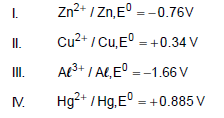Solution:
QUESTION: 20

Which of the following is not a correct statement about electrochemical series of reduction potentials?

Solution: